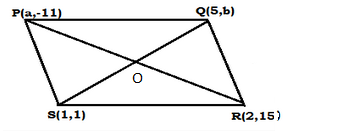# If the points P(a, −11), Q(5, b), R(2, 15) and S(1, 1) are the vertices of a parallelogram PQRS,Question:

If the points P(a, −11), Q(5, b), R(2, 15) and S(1, 1) are the vertices of a parallelogram PQRS, find the values of a and b.

Solution:

The points are P(a, −11), Q(5, b), R(2, 15) and S(1, 1).Join PR and QS, intersecting at O.
We know that the diagonals of a parallelogram bisect each other.
Therefore, O is the midpoint of PR as well as QS.

Midpoint of $P R=\left(\frac{a+2}{2}, \frac{-11+15}{2}\right)=\left(\frac{a+2}{2}, \frac{4}{2}\right)=\left(\frac{a+2}{2}, 2\right)$

Midpoint of $Q S=\left(\frac{5+1}{2}, \frac{b+1}{2}\right)=\left(\frac{6}{2}, \frac{b+1}{2}\right)=\left(3, \frac{b+1}{2}\right)$

Therefore, $\frac{a+2}{2}=3, \frac{b+1}{2}=2$

$\Rightarrow a+2=6, b+1=4$

$\Rightarrow a=6-2, b=4-1$

$\Rightarrow a=4$ and $b=3$